# Varignon Investigation

• Use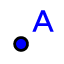create 4 random points (A,B,C,D).
• Use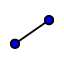to connect the points and form a quadrilateral.
• Use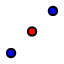to find the midpoint of each side.
• Use ﻿to connect the four midpoints.
• Useto move points A, B, C, or D.

## What type of quadrilaterals are formed?

Use the applet below to investigate the type of quadrilateral that is formed by the midpoints of various figures.
• Follow the same steps as above to create the Varignon quadrilateral for each shape below.
• You can move the green points.
• What type of Varignon quadrilateral is formed by each shape?
• Use the,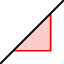, and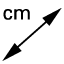tools to verify your answers.